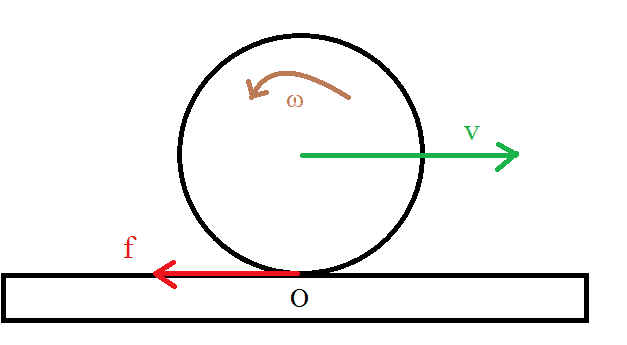# Rolling without slipping taking the contact point as pivot

• Soren4

#### Soren4

I'm confused about this rolling without (or better with) slipping situation. Suppose to have a disk with initial velocity ##v## and angular velocity ##\omega##. The motion is to the right but the angular velocity is counterclockwise.There are no forces acting on the disk besides the kinetic friction##\mathbf{f}##.

Things are ok if I take as pivot point the center of mass.
$$\{\begin{matrix} - \mathbf{f} = m\mathbf{a_{CM}}\\ - \mathbf{r} \times \mathbf{f} =I_{cm} \mathbf{\alpha} \end{matrix}\tag{1}$$

But if I take the point ##O## on the ground, then the kinetic friction has zero torque.

$$\{\begin{matrix} - \mathbf{f} = m\mathbf{a_{CM}}\\ 0 =I_{O} \mathbf{\alpha} \end{matrix}\tag{2}$$

I assumed that the angular velocity (and so $\alpha$) is the same it I take as pivot the center of mass or the point##O##.

If this is the case than parallel axis theorem can be used and $$I_O=I_{cm}+m \mathbf{r}^2$$

But there is a contradiction since I get ##\alpha=0## from ##(2)## and ##\alpha\neq0## from##(1)##.

How can that be? Maybe##\alpha## is not the same in the two cases?

Last edited:
I'm confused about this rolling without slipping situation. Suppose to have a disk with initial velocity vvv and angular velocity ωω\omega. The motion is to the right but the angular velocity is counterclockwise.

i fail to see a counter clockwise angular velocity when the disk is moving to the right side.

•Soren4
i fail to see a counter clockwise angular velocity when the disk is moving to the right side.
I'm sorry the disk is actually slipping, I called this rolling without slippping problem, because usually in these problems you have to find the conditions for rolling without slipping indeed.

Just ignore it, the disk is slipping, of course

I'm sorry the disk is actually slipping, I called this rolling without slippping problem, because usually in these problems you have to find the conditions for rolling without slipping indeed.

Just ignore it, the disk is slipping, of course

if the disk is slipping only ,then there is no rolling motion;

then the equations for the motion of center of mass only and
the frictional force will be slowly decelerating the motion - no rotation of the disk.

if you consider both rolling and slipping combined then only the torque due to friction will operate about an axis passing through center of mass,

If the disk is sliding, O is not a pivot point, and could not be used as a basis for parallel axis method. The bottom point on the disk would be a pivot point, but it's sliding and decelerating.

You could consider the contact point of the sliding disk as a pivot point, but it's an accelerating (decelerating) frame of reference, so there would be a fictitious force effectively applied to the center of mass of the disk, related to the acceleration (deceleration) of the disk.

Last edited: